## Excel Tutorial

 ELE 343 Electronics II This tutorial demonstrates the use of EXCEL to Evaluate mathematical expressions. Plot data Extract model parameters from data Evaluating Formulas The excel sheet data shown in Figure 1 represents transistor currents. To calculate the transistor beta (Ic/Ib) at different transistor currents; Select cell E6 as shown. Click on the equal sign above the spread sheet area. As shown, type "D6/C6" in the box following the equal sign. D6 and C6 are the cells containing Ic and Ib. Hit "ENTER" and their quotient will appear in the selected cell, E6. Place the cursor over the square dot in the lower right corner of the selected cell, E6. The square dot becomes a cross. Drag the cross down the column to cell E14. This copies the formula to these cells. The correct Ib and Ic are referenced to produce the appropriate beta values. More complex formulas can be evaluated.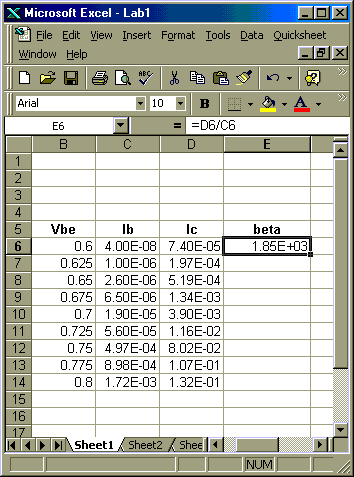Figure 1
 Plotting Data Problem:   Plot the log of Ib and Ic vs Vbe Solution: Select the data to be plotted as shown in Figure 2. Click on the chart icon,shown on the EXCEL sheet in Figure 2.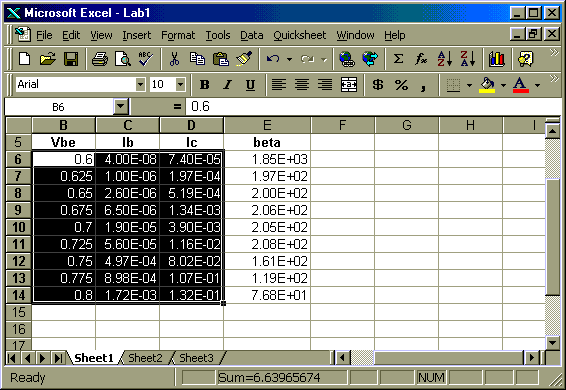Figure 2
 In the titles option window of the chart wizard, fill in the desired graph and axis titles as shown in Figure 3.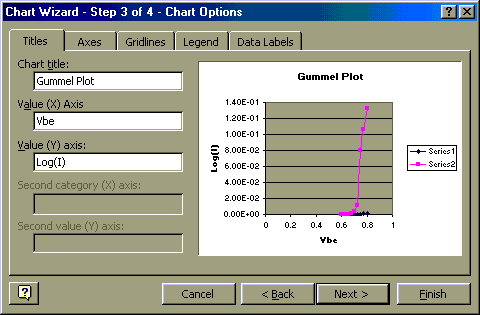Figure 3

1. Either select the axis option of the chart wizard or finish the plot and then select the vertical axis. With the vertical axis selected, click the right mouse button. In the window that opens, select "format axis". The window shown in Figure 4 results.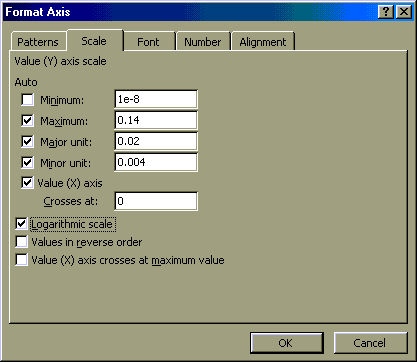Figure 4   When selecting a logarithmic axis, be sure zero or negative values are not included. Select a minimum greater than zero and check "logarithmic scale".

Fitting Data to a Formula

A formula representation of the collector current data can be obtained.

1. Select the curve representing Ic in the graph.

2. Click the right mouse button.

3. In the window that comes up, select "Add Trendline"

4. Select   Type > exponential

5. Select   Option > display equation on chart
As shown below, eliminate data that does not look like it is on a straight line in the gummel plot. This results in a more accurate formula over a smaller Vbe range.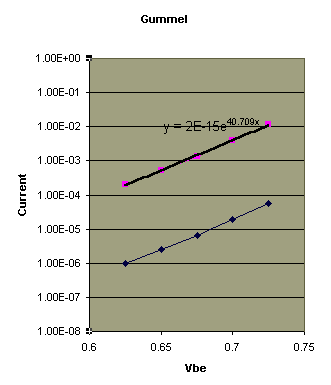EXCEL formula shown in graph:     Ic = 2E-15 e40.709 Vbe

SPICE uses a formula
Ic = IS eVbe/(NF Vt)
where IS and NF are SPICE model parameters and Vt = KT/q = 0.0259V at T=300 degrees Kelvin.

Matching the EXCEL and SPICE formulas for Ic allows two important SPICE model parameters to be determined.

IS = 2E-15
NF = 0.95